# Spark Tutorial: Learning Apache Spark¶

#### The following transformations will be covered:¶

• #### map(), mapPartitions(), mapPartitionsWithIndex(), filter(), flatMap(), reduceByKey(), groupByKey() #### The following actions will be covered:
• #### first(), take(), takeSample(), takeOrdered(), collect(), count(), countByValue(), reduce(), top() #### Also covered:
• #### cache(), unpersist(), id(), setName() #### Note that, for reference, you can look up the details of these methods in Spark's Python API

### Part 1: Basic notebook usage and Python integration ¶

#### (1a) Notebook usage¶

In [ ]:
# This is a Python cell. You can run normal Python code here...
print 'The sum of 1 and 1 is {0}'.format(1+1)

In [ ]:
# Here is another Python cell, this time with a variable (x) declaration and an if statement:
x = 42
if x > 40:
print 'The sum of 1 and 2 is {0}'.format(1+2)


#### As you work through a notebook it is important that you run all of the code cells. The notebook is stateful, which means that variables and their values are retained until the notebook is detached (in Databricks Cloud) or the kernel is restarted (in IPython notebooks). If you do not run all of the code cells as you proceed through the notebook, your variables will not be properly initialized and later code might fail. You will also need to rerun any cells that you have modified in order for the changes to be available to other cells.¶

In [ ]:
# This cell relies on x being defined already.
# If we didn't run the cells from part (1a) this code would fail.
print x * 2


#### We can import standard Python libraries (modules) the usual way. An import statement will import the specified module. In this tutorial and future labs, we will provide any imports that are necessary.¶

In [ ]:
# Import the regular expression library
import re
m = re.search('(?<=abc)def', 'abcdef')
m.group(0)

In [ ]:
# Import the datetime library
import datetime
print 'This was last run on: {0}'.format(datetime.datetime.now())


### Part 2: An introduction to using Apache Spark with the Python pySpark API running in the browser¶

#### The diagram below shows an example cluster, where the cores allocated for an application are outlined in purple.¶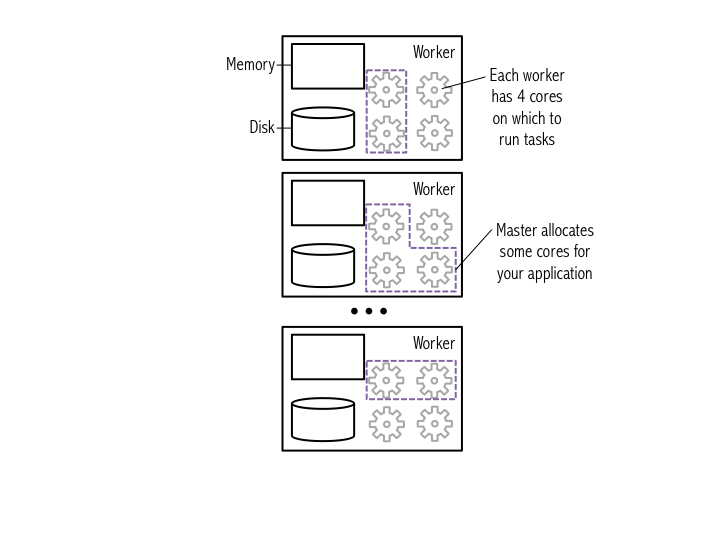#### Try printing out sc to see its type.¶

In [ ]:
# Display the type of the Spark Context sc
type(sc)


#### You can use Python's dir() function to get a list of all the attributes (including methods) accessible through the sc object.¶

In [ ]:
# List sc's attributes
dir(sc)


#### Alternatively, you can use Python's help() function to get an easier to read list of all the attributes, including examples, that the sc object has.¶

In [ ]:
# Use help to obtain more detailed information
help(sc)

In [ ]:
# After reading the help we've decided we want to use sc.version to see what version of Spark we are running
sc.version

In [ ]:
# Help can be used on any Python object
help(map)


### Part 3: Using RDDs and chaining together transformations and actions¶

#### We will perform several exercises to obtain a better understanding of RDDs:¶

• ##### Create a Python collection of 10,000 integers
• ##### Create a Spark base RDD from that collection
• ##### Subtract one from each value using map
• ##### Perform action collect to view results
• ##### Perform action count to view counts
• ##### Apply transformation filter and view results with collect
• ##### Learn about lambda functions
• ##### Explore how lazy evaluation works and the debugging challenges that it introduces

#### We will use the xrange() function to create a list() of integers. xrange() only generates values as they are needed. This is different from the behavior of range() which generates the complete list upon execution. Because of this xrange() is more memory efficient than range(), especially for large ranges.¶

In [ ]:
data = xrange(1, 10001)

In [ ]:
# Data is just a normal Python list
# Obtain data's first element
data

In [ ]:
# We can check the size of the list using the len() function
len(data)


#### The figure below illustrates how Spark breaks a list of data entries into partitions that are each stored in memory on a worker.¶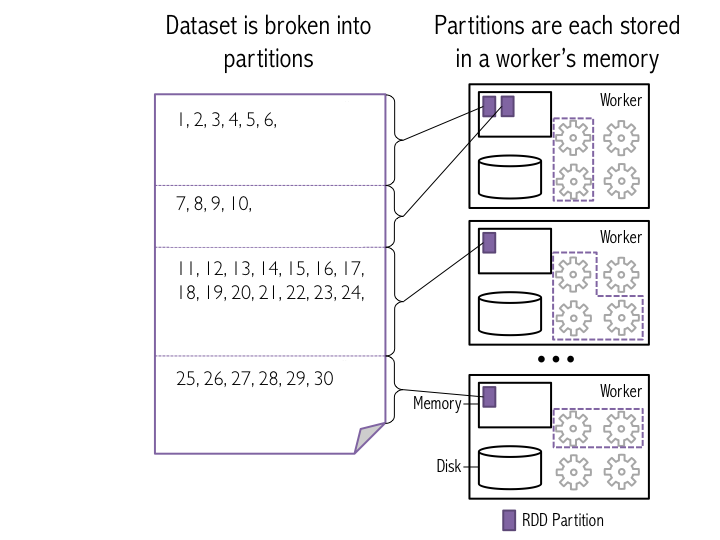#### After we generate RDDs, we can view them in the "Storage" tab of the web UI. You'll notice that new datasets are not listed until Spark needs to return a result due to an action being executed. This feature of Spark is called "lazy evaluation". This allows Spark to avoid performing unnecessary calculations.¶

In [ ]:
# Parallelize data using 8 partitions
# This operation is a transformation of data into an RDD
# Spark uses lazy evaluation, so no Spark jobs are run at this point
xrangeRDD = sc.parallelize(data, 8)

In [ ]:
# Let's view help on parallelize
help(sc.parallelize)

In [ ]:
# Let's see what type sc.parallelize() returned
print 'type of xrangeRDD: {0}'.format(type(xrangeRDD))

# How about if we use a range
dataRange = range(1, 10001)
rangeRDD = sc.parallelize(dataRange, 8)
print 'type of dataRangeRDD: {0}'.format(type(rangeRDD))

In [ ]:
# Each RDD gets a unique ID
print 'xrangeRDD id: {0}'.format(xrangeRDD.id())
print 'rangeRDD id: {0}'.format(rangeRDD.id())

In [ ]:
# We can name each newly created RDD using the setName() method
xrangeRDD.setName('My first RDD')

In [ ]:
# Let's view the lineage (the set of transformations) of the RDD using toDebugString()
print xrangeRDD.toDebugString()

In [ ]:
# Let's use help to see what methods we can call on this RDD
help(xrangeRDD)

In [ ]:
# Let's see how many partitions the RDD will be split into by using the getNumPartitions()
xrangeRDD.getNumPartitions()


#### map(f), the most common Spark transformation, is one such example: it applies a function f to each item in the dataset, and outputs the resulting dataset. When you run map() on a dataset, a single stage of tasks is launched. A stage is a group of tasks that all perform the same computation, but on different input data. One task is launched for each partitition, as shown in the example below. A task is a unit of execution that runs on a single machine. When we run map(f) within a partition, a new task applies f to all of the entries in a particular partition, and outputs a new partition. In this example figure, the dataset is broken into four partitions, so four map() tasks are launched.¶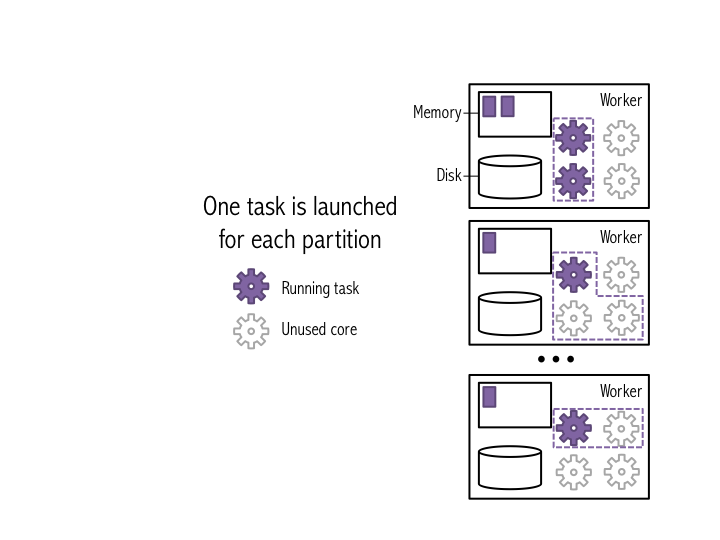#### The figure below shows how this would work on the smaller data set from the earlier figures. Note that one task is launched for each partition.¶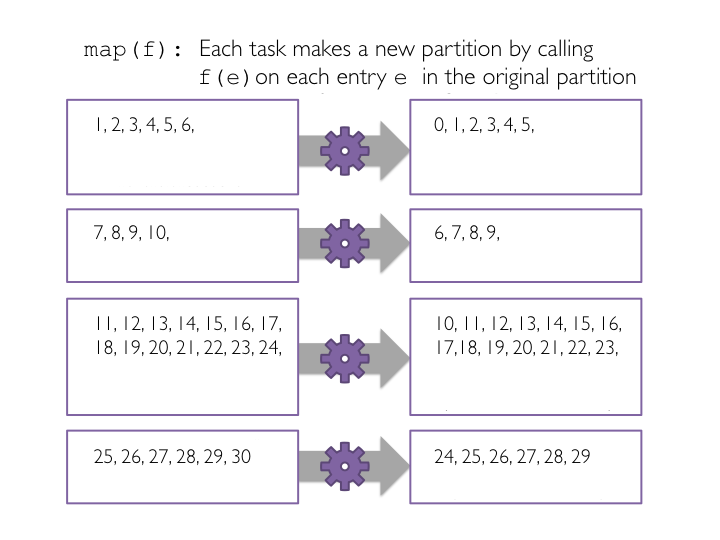#### Now we will use map() to subtract one from each value in the base RDD we just created. First, we define a Python function called sub() that will subtract one from the input integer. Second, we will pass each item in the base RDD into a map() transformation that applies the sub() function to each element. And finally, we print out the RDD transformation hierarchy using toDebugString().¶

In [ ]:
# Create sub function to subtract 1
def sub(value):
""""Subtracts one from value.

Args:
value (int): A number.

Returns:
int: value minus one.
"""
return (value - 1)

# Transform xrangeRDD through map transformation using sub function
# Because map is a transformation and Spark uses lazy evaluation, no jobs, stages,
# or tasks will be launched when we run this code.
subRDD = xrangeRDD.map(sub)

# Let's see the RDD transformation hierarchy
print subRDD.toDebugString()


#### In this example, the dataset is broken into four partitions, so four collect() tasks are launched. Each task collects the entries in its partition and sends the result to the SparkContext, which creates a list of the values, as shown in the figure below.¶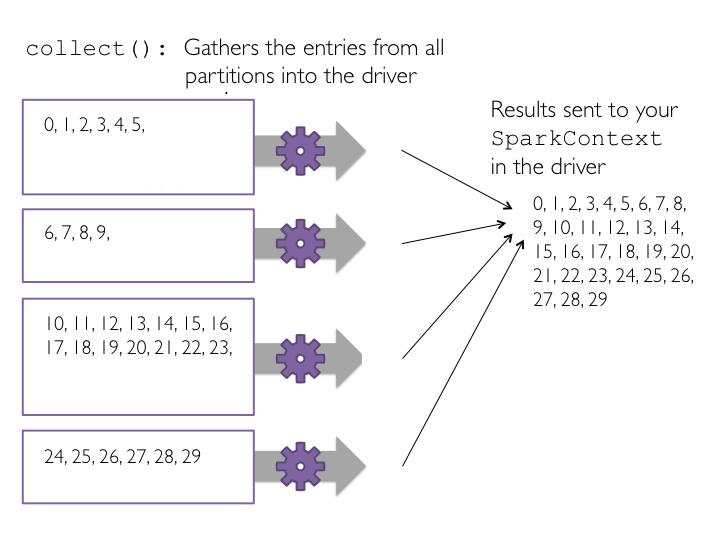#### Now let's run collect() on subRDD.¶

In [ ]:
# Let's collect the data
print subRDD.collect()


#### Each task counts the entries in its partition and sends the result to your SparkContext, which adds up all of the counts. The figure below shows what would happen if we ran count() on a small example dataset with just four partitions.¶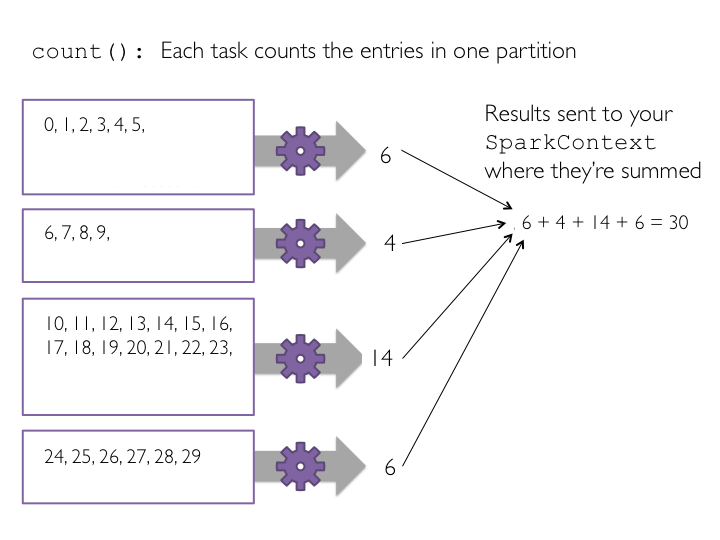In [ ]:
print xrangeRDD.count()
print subRDD.count()


#### The figure below shows how this would work on the small four-partition dataset.¶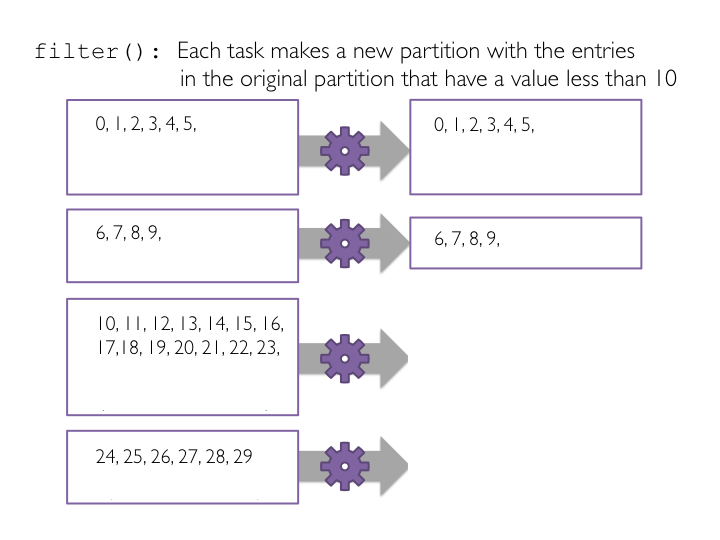#### To view the filtered list of elements less than ten, we need to create a new list on the driver from the distributed data on the executor nodes. We use the collect() method to return a list that contains all of the elements in this filtered RDD to the driver program.¶

In [ ]:
# Define a function to filter a single value
def ten(value):
"""Return whether value is below ten.

Args:
value (int): A number.

Returns:
bool: Whether value is less than ten.
"""
if (value < 10):
return True
else:
return False
# The ten function could also be written concisely as: def ten(value): return value < 10

# Pass the function ten to the filter transformation
# Filter is a transformation so no tasks are run
filteredRDD = subRDD.filter(ten)

# View the results using collect()
# Collect is an action and triggers the filter transformation to run
print filteredRDD.collect()


### Part 4: Lambda Functions ¶

#### Here, instead of defining a separate function for the filter() transformation, we will use an inline lambda() function.¶

In [ ]:
lambdaRDD = subRDD.filter(lambda x: x < 10)
lambdaRDD.collect()

In [ ]:
# Let's collect the even values less than 10
evenRDD = lambdaRDD.filter(lambda x: x % 2 == 0)
evenRDD.collect()


### Part 5: Additional RDD actions ¶

#### The reduce() action reduces the elements of a RDD to a single value by applying a function that takes two parameters and returns a single value. The function should be commutative and associative, as reduce() is applied at the partition level and then again to aggregate results from partitions. If these rules don't hold, the results from reduce() will be inconsistent. Reducing locally at partitions makes reduce() very efficient.¶

In [ ]:
# Let's get the first element
print filteredRDD.first()
# The first 4
print filteredRDD.take(4)
# Note that it is ok to take more elements than the RDD has
print filteredRDD.take(12)

In [ ]:
# Retrieve the three smallest elements
print filteredRDD.takeOrdered(3)
# Retrieve the five largest elements
print filteredRDD.top(5)

In [ ]:
# Pass a lambda function to takeOrdered to reverse the order
filteredRDD.takeOrdered(4, lambda s: -s)

In [ ]:
# Obtain Python's add function
# Efficiently sum the RDD using reduce
# Sum using reduce with a lambda function
print filteredRDD.reduce(lambda a, b: a + b)
# Note that subtraction is not both associative and commutative
print filteredRDD.reduce(lambda a, b: a - b)
print filteredRDD.repartition(4).reduce(lambda a, b: a - b)
print filteredRDD.repartition(4).reduce(lambda a, b: a + b)


#### The countByValue() action returns the count of each unique value in the RDD as a dictionary that maps values to counts.¶

In [ ]:
# takeSample reusing elements
print filteredRDD.takeSample(withReplacement=True, num=6)
# takeSample without reuse
print filteredRDD.takeSample(withReplacement=False, num=6)

In [ ]:
# Set seed for predictability
print filteredRDD.takeSample(withReplacement=False, num=6, seed=500)
# Try reruning this cell and the cell above -- the results from this cell will remain constant
# Use ctrl-enter to run without moving to the next cell

In [ ]:
# Create new base RDD to show countByValue
repetitiveRDD = sc.parallelize([1, 2, 3, 1, 2, 3, 1, 2, 1, 2, 3, 3, 3, 4, 5, 4, 6])
print repetitiveRDD.countByValue()


### Part 6: Additional RDD transformations ¶

#### To demonstrate flatMap(), we will first emit a word along with its plural, and then a range that grows in length with each subsequent operation.¶

In [ ]:
# Let's create a new base RDD to work from
wordsList = ['cat', 'elephant', 'rat', 'rat', 'cat']
wordsRDD = sc.parallelize(wordsList, 4)

# Use map
singularAndPluralWordsRDDMap = wordsRDD.map(lambda x: (x, x + 's'))
# Use flatMap
singularAndPluralWordsRDD = wordsRDD.flatMap(lambda x: (x, x + 's'))

# View the results
print singularAndPluralWordsRDDMap.collect()
print singularAndPluralWordsRDD.collect()
# View the number of elements in the RDD
print singularAndPluralWordsRDDMap.count()
print singularAndPluralWordsRDD.count()

In [ ]:
simpleRDD = sc.parallelize([2, 3, 4])
print simpleRDD.map(lambda x: range(1, x)).collect()
print simpleRDD.flatMap(lambda x: range(1, x)).collect()


#### Look at the diagram below to understand how reduceByKey works. Notice how pairs on the same machine with the same key are combined (by using the lamdba function passed into reduceByKey) before the data is shuffled. Then the lamdba function is called again to reduce all the values from each partition to produce one final result.¶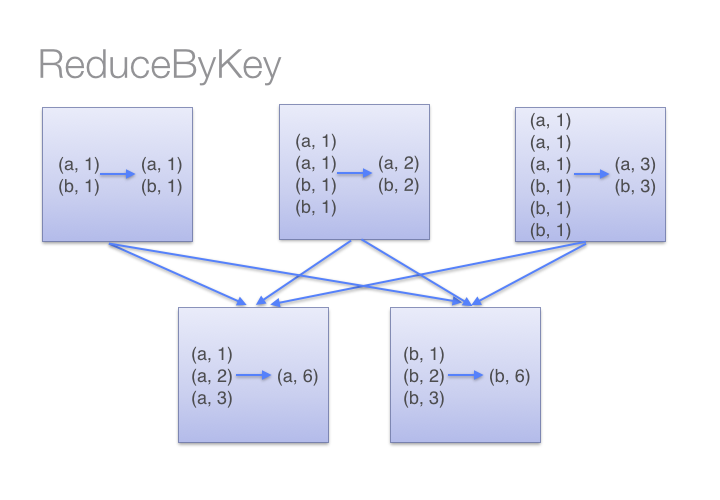#### To determine which machine to shuffle a pair to, Spark calls a partitioning function on the key of the pair. Spark spills data to disk when there is more data shuffled onto a single executor machine than can fit in memory. However, it flushes out the data to disk one key at a time, so if a single key has more key-value pairs than can fit in memory an out of memory exception occurs. This will be more gracefully handled in a later release of Spark so that the job can still proceed, but should still be avoided. When Spark needs to spill to disk, performance is severely impacted.¶#### Here are more transformations to prefer over groupByKey():¶

• #### combineByKey() can be used when you are combining elements but your return type differs from your input value type.
• #### foldByKey() merges the values for each key using an associative function and a neutral "zero value". #### Now let's go through a simple groupByKey() and reduceByKey() example.
In [ ]:
pairRDD = sc.parallelize([('a', 1), ('a', 2), ('b', 1)])
# mapValues only used to improve format for printing
print pairRDD.groupByKey().mapValues(lambda x: list(x)).collect()

# Different ways to sum by key
print pairRDD.groupByKey().map(lambda (k, v): (k, sum(v))).collect()
# Using mapValues, which is recommended when they key doesn't change
print pairRDD.groupByKey().mapValues(lambda x: sum(x)).collect()
# reduceByKey is more efficient / scalable


#### The mapPartitionsWithIndex() transformation uses a function that takes in a partition index (think of this like the partition number) and an iterator (to the items in that specific partition). For every partition (index, iterator) pair, the function returns a tuple of the same partition index number and an iterator of the transformed items in that partition.¶

In [ ]:
# mapPartitions takes a function that takes an iterator and returns an iterator
print wordsRDD.collect()
itemsRDD = wordsRDD.mapPartitions(lambda iterator: [','.join(iterator)])
print itemsRDD.collect()

In [ ]:
itemsByPartRDD = wordsRDD.mapPartitionsWithIndex(lambda index, iterator: [(index, list(iterator))])
# We can see that three of the (partitions) workers have one element and the fourth worker has two
# elements, although things may not bode well for the rat...
print itemsByPartRDD.collect()
# Rerun without returning a list (acts more like flatMap)
itemsByPartRDD = wordsRDD.mapPartitionsWithIndex(lambda index, iterator: (index, list(iterator)))
print itemsByPartRDD.collect()


### Part 7: Caching RDDs and storage options ¶

#### You can check if an RDD is cached by using the is_cached attribute, and you can see your cached RDD in the "Storage" section of the Spark web UI. If you click on the RDD's name, you can see more information about where the RDD is stored.¶

In [ ]:
# Name the RDD
filteredRDD.setName('My Filtered RDD')
# Cache the RDD
filteredRDD.cache()
# Is it cached
print filteredRDD.is_cached


#### Advanced: Spark provides many more options for managing how RDDs are stored in memory or even saved to disk. You can explore the API for RDD's persist() operation using Python's help() command. The persist() operation, optionally, takes a pySpark StorageLevel object.¶

In [ ]:
# Note that toDebugString also provides storage information
print filteredRDD.toDebugString()

In [ ]:
# If we are done with the RDD we can unpersist it so that its memory can be reclaimed
filteredRDD.unpersist()
# Storage level for a non cached RDD
print filteredRDD.getStorageLevel()
filteredRDD.cache()
# Storage level for a cached RDD
print filteredRDD.getStorageLevel()


### Part 8: Debugging Spark applications and lazy evaluation ¶

#### The filter() method will not be executed until an action operation is invoked on the RDD. We will perform an action by using the collect() method to return a list that contains all of the elements in this RDD.¶

In [ ]:
def brokenTen(value):
"""Incorrect implementation of the ten function.

Note:
The if statement checks an undefined variable val instead of value.

Args:
value (int): A number.

Returns:
bool: Whether value is less than ten.

Raises:
NameError: The function references val, which is not available in the local or global
namespace, so a NameError is raised.
"""
if (val < 10):
return True
else:
return False

brokenRDD = subRDD.filter(brokenTen)

In [ ]:
# Now we'll see the error
brokenRDD.collect()


#### Scroll through the output "Py4JJavaError Traceback (most recent call last)" part of the cell and first you will see that the line that generated the error is the collect() method line. There is nothing wrong with this line. However, it is an action and that caused other methods to be executed. Continue scrolling through the Traceback and you will see the following error line:¶

NameError: global name 'val' is not defined


#### As you are learning Spark, I recommend that you write your code in the form:¶

RDD.transformation1()
RDD.action1()
RDD.transformation2()
RDD.action2()


#### Once you become more experienced with Spark, you can write your code with the form:¶

RDD.transformation1().transformation2().action()


#### We can also use lambda() functions instead of separately defined functions when their use improves readability and conciseness.¶

In [ ]:
# Cleaner code through lambda use
subRDD.filter(lambda x: x < 10).collect()

In [ ]:
# Even better by moving our chain of operators into a single line.
sc.parallelize(data).map(lambda y: y - 1).filter(lambda x: x < 10).collect()


#### To make the expert coding style more readable, enclose the statement in parentheses and put each method, transformation, or action on a separate line.¶

In [ ]:
# Final version
(sc
.parallelize(data)
.map(lambda y: y - 1)
.filter(lambda x: x < 10)
.collect())